# 9.1 Sequences and their notations  (Page 7/15)

 Page 7 / 15

$\left(\frac{12}{6}\right)!$

$\frac{12!}{6!}$

$665,280$

$\frac{100!}{99!}$

For the following exercises, write the first four terms of the sequence.

${a}_{n}=\frac{n!}{{n}^{\text{2}}}$

First four terms: $1,\frac{1}{2},\frac{2}{3},\frac{3}{2}$

${a}_{n}=\frac{3\cdot n!}{4\cdot n!}$

${a}_{n}=\frac{n!}{{n}^{2}-n-1}$

First four terms: $-1,2,\frac{6}{5},\frac{24}{11}$

${a}_{n}=\frac{100\cdot n}{n\left(n-1\right)!}$

## Graphical

For the following exercises, graph the first five terms of the indicated sequence

${a}_{n}=\frac{{\left(-1\right)}^{n}}{n}+n$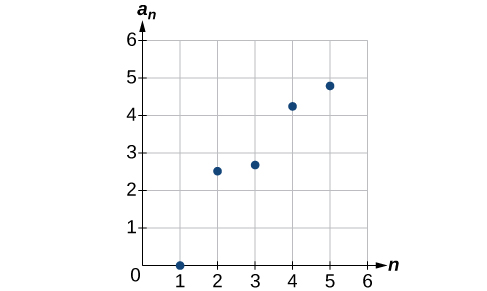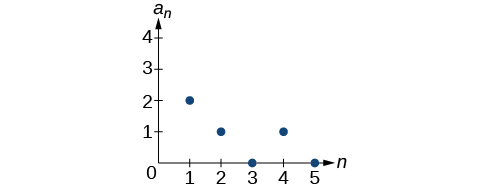${a}_{n}=\frac{\left(n+1\right)!}{\left(n-1\right)!}$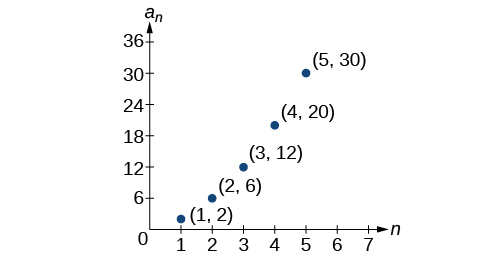For the following exercises, write an explicit formula for the sequence using the first five points shown on the graph.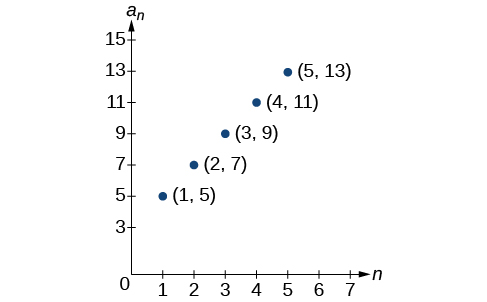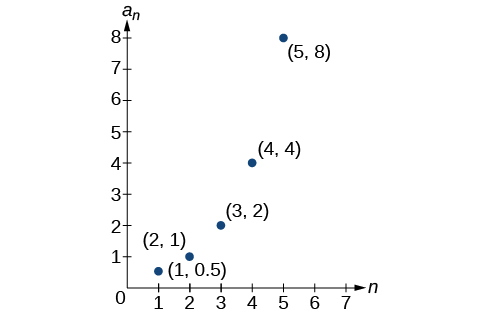${a}_{n}={2}^{n-2}$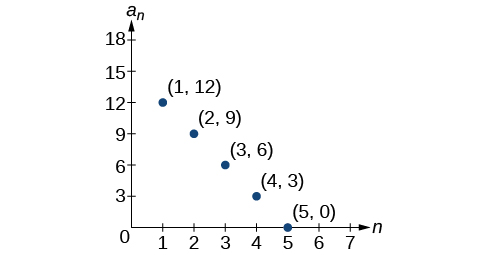For the following exercises, write a recursive formula for the sequence using the first five points shown on the graph.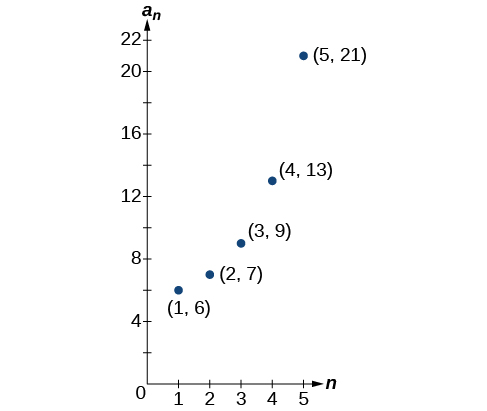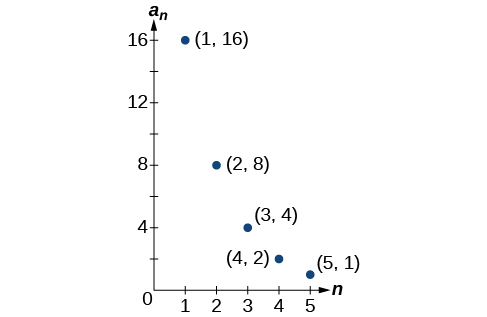## Technology

Follow these steps to evaluate a sequence defined recursively using a graphing calculator:

• On the home screen, key in the value for the initial term $\text{\hspace{0.17em}}{a}_{1}\text{\hspace{0.17em}}$ and press [ENTER] .
• Enter the recursive formula by keying in all numerical values given in the formula, along with the key strokes [2ND] ANS for the previous term $\text{\hspace{0.17em}}{a}_{n-1}.\text{\hspace{0.17em}}$ Press [ENTER] .
• Continue pressing [ENTER] to calculate the values for each successive term.

For the following exercises, use the steps above to find the indicated term or terms for the sequence.

Find the first five terms of the sequence Use the> Frac feature to give fractional results.

First five terms: $\frac{29}{37},\frac{152}{111},\frac{716}{333},\frac{3188}{999},\frac{13724}{2997}$

Find the 15 th term of the sequence

Find the first five terms of the sequence

First five terms: $2,3,5,17,65537$

Find the first ten terms of the sequence

Find the tenth term of the sequence

${a}_{10}=7,257,600$

Follow these steps to evaluate a finite sequence defined by an explicit formula. Using a TI-84, do the following.

• In the home screen, press [2ND] LIST .
• Scroll over to OPS and choose “seq(” from the dropdown list. Press [ENTER] .
• In the line headed “Expr:” type in the explicit formula, using the $\text{\hspace{0.17em}}\left[\text{X,T},\theta ,n\right]\text{\hspace{0.17em}}$ button for $\text{\hspace{0.17em}}n$
• In the line headed “Variable:” type in the variable used on the previous step.
• In the line headed “start:” key in the value of $\text{\hspace{0.17em}}n\text{\hspace{0.17em}}$ that begins the sequence.
• In the line headed “end:” key in the value of $\text{\hspace{0.17em}}n\text{\hspace{0.17em}}$ that ends the sequence.
• Press [ENTER] 3 times to return to the home screen. You will see the sequence syntax on the screen. Press [ENTER] to see the list of terms for the finite sequence defined. Use the right arrow key to scroll through the list of terms.

Using a TI-83, do the following.

• In the home screen, press [2ND] LIST .
• Scroll over to OPS and choose “seq(” from the dropdown list. Press [ENTER] .
• Enter the items in the order “Expr” , “Variable” , “start” , “end” separated by commas. See the instructions above for the description of each item.
• Press [ENTER] to see the list of terms for the finite sequence defined. Use the right arrow key to scroll through the list of terms.

For the following exercises, use the steps above to find the indicated terms for the sequence. Round to the nearest thousandth when necessary.

List the first five terms of the sequence ${a}_{n}=-\frac{28}{9}n+\frac{5}{3}.$

List the first six terms of the sequence

First six terms: $0.042,0.146,0.875,2.385,4.708$

List the first five terms of the sequence ${a}_{n}=\frac{15n\cdot {\left(-2\right)}^{n-1}}{47}$

List the first four terms of the sequence ${a}_{n}={5.7}^{n}+0.275\left(n-1\right)!$

First four terms: $5.975,32.765,185.743,1057.25,6023.521$

List the first six terms of the sequence ${a}_{n}=\frac{n!}{n}.$

## Extensions

Consider the sequence defined by ${a}_{n}=-6-8n.$ Is ${a}_{n}=-421$ a term in the sequence? Verify the result.

If $\text{\hspace{0.17em}}{a}_{n}=-421\text{\hspace{0.17em}}$ is a term in the sequence, then solving the equation $-421=-6-8n$ for $n$ will yield a non-negative integer. However, if $\text{\hspace{0.17em}}-421=-6-8n,\text{\hspace{0.17em}}$ then $n=51.875$ so ${a}_{n}=-421$ is not a term in the sequence.

What term in the sequence ${a}_{n}=\frac{{n}^{2}+4n+4}{2\left(n+2\right)}$ has the value $41?$ Verify the result.

Find a recursive formula for the sequence ( Hint : find a pattern for $\text{\hspace{0.17em}}{a}_{n}\text{\hspace{0.17em}}$ based on the first two terms.)

${a}_{1}=1,{a}_{2}=0,{a}_{n}={a}_{n-1}-{a}_{n-2}$

Calculate the first eight terms of the sequences ${a}_{n}=\frac{\left(n+2\right)!}{\left(n-1\right)!}$ and ${b}_{n}={n}^{3}+3{n}^{2}+2n,$ and then make a conjecture about the relationship between these two sequences.

Prove the conjecture made in the preceding exercise.

$\frac{\left(n+2\right)!}{\left(n-1\right)!}=\frac{\left(n+2\right)·\left(n+1\right)·\left(n\right)·\left(n-1\right)·...·3·2·1}{\left(n-1\right)·...·3·2·1}=n\left(n+1\right)\left(n+2\right)={n}^{3}+3{n}^{2}+2n$

show that the set of all natural number form semi group under the composition of addition
what is the meaning
Dominic
explain and give four Example hyperbolic function
_3_2_1
felecia
⅗ ⅔½
felecia
_½+⅔-¾
felecia
The denominator of a certain fraction is 9 more than the numerator. If 6 is added to both terms of the fraction, the value of the fraction becomes 2/3. Find the original fraction. 2. The sum of the least and greatest of 3 consecutive integers is 60. What are the valu
1. x + 6 2 -------------- = _ x + 9 + 6 3 x + 6 3 ----------- x -- (cross multiply) x + 15 2 3(x + 6) = 2(x + 15) 3x + 18 = 2x + 30 (-2x from both) x + 18 = 30 (-18 from both) x = 12 Test: 12 + 6 18 2 -------------- = --- = --- 12 + 9 + 6 27 3
Pawel
2. (x) + (x + 2) = 60 2x + 2 = 60 2x = 58 x = 29 29, 30, & 31
Pawel
ok
Ifeanyi
on number 2 question How did you got 2x +2
Ifeanyi
combine like terms. x + x + 2 is same as 2x + 2
Pawel
x*x=2
felecia
2+2x=
felecia
×/×+9+6/1
Debbie
Q2 x+(x+2)+(x+4)=60 3x+6=60 3x+6-6=60-6 3x=54 3x/3=54/3 x=18 :. The numbers are 18,20 and 22
Naagmenkoma
Mark and Don are planning to sell each of their marble collections at a garage sale. If Don has 1 more than 3 times the number of marbles Mark has, how many does each boy have to sell if the total number of marbles is 113?
Mark = x,. Don = 3x + 1 x + 3x + 1 = 113 4x = 112, x = 28 Mark = 28, Don = 85, 28 + 85 = 113
Pawel
how do I set up the problem?
what is a solution set?
Harshika
find the subring of gaussian integers?
Rofiqul
hello, I am happy to help!
Abdullahi
hi mam
Mark
find the value of 2x=32
divide by 2 on each side of the equal sign to solve for x
corri
X=16
Michael
Want to review on complex number 1.What are complex number 2.How to solve complex number problems.
Beyan
yes i wantt to review
Mark
16
Makan
x=16
Makan
use the y -intercept and slope to sketch the graph of the equation y=6x
how do we prove the quadratic formular
Darius
hello, if you have a question about Algebra 2. I may be able to help. I am an Algebra 2 Teacher
thank you help me with how to prove the quadratic equation
Seidu
may God blessed u for that. Please I want u to help me in sets.
Opoku
what is math number
4
Trista
x-2y+3z=-3 2x-y+z=7 -x+3y-z=6
can you teacch how to solve that🙏
Mark
Solve for the first variable in one of the equations, then substitute the result into the other equation. Point For: (6111,4111,−411)(6111,4111,-411) Equation Form: x=6111,y=4111,z=−411x=6111,y=4111,z=-411
Brenna
(61/11,41/11,−4/11)
Brenna
x=61/11 y=41/11 z=−4/11 x=61/11 y=41/11 z=-4/11
Brenna
Need help solving this problem (2/7)^-2
x+2y-z=7
Sidiki
what is the coefficient of -4×
-1
Shedrak

#### Get Jobilize Job Search Mobile App in your pocket Now!ByBy OpenStaxBy OpenStaxBy Szilárd JankóBy Madison ChristianBy OpenStaxBy OpenStaxBy Edgar DelgadoBy OpenStaxBy OpenStaxBy Joli Julianna